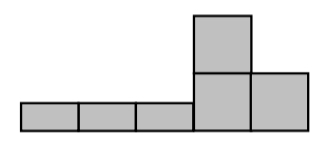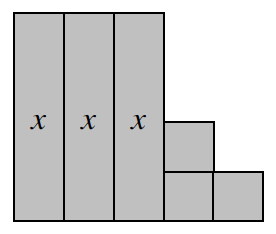### Home > CC2 > Chapter 5 > Lesson 5.2.3 > Problem5-51

5-51.

On your paper, sketch the algebra tile shape shown at right. Write expressions for the area and perimeter of the shape. Then calculate the area and perimeter of the shape for each $x\text{-value}$.

Add the areas of all six tiles to find the shape's total area.

Area: $3x+3$

Add the lengths of all the sides of the shape to find the perimeter.

Try labeling all the sides first.

Perimeter: $2x+10$

1. $x=9$ cm

Substitute $9$ cm for $x$ in each expression.

$A=3(9)+3$
$P=2(9)+10$
Use the Order of Operations to simplify and calculate the answer.

$A=30$ sq cm
$P=28$ cm

2. $x=0.5$ cm Hint: Draw a diagram. Does your perimeter expression work?$A=4.5$ sq cm
$P=14$ cm

3. $x=15$ cm

See part (a).